# transform filters

We often want to repeat the same CQL filter but just changing some things: we might want to change the side to move, or shift all the pieces one square up the board.

Transform filters are a group of filters that allow for this behavior. The transform filters are `flip`, `flipcolor`, ....

A transform filter always has this syntax:

`  transform_filter argument`

Here, transform_filter is the name of the transform filter (e.g., `flip`, `flipcolor`, `shift`). The argument is any filter.

Each transform filter has an associated with a set of basic transforms. A basic transform transforms a filter into a new filter. We say a basic transform transforms a filter to create a transformed filter.

Consider a transform filter T with an associated set of basic transforms

`  T1, T2,...,Tn`

There are two cases.

If argument is a set filter, then `T argument` is replaced in the CQL file with:

```  {T1 argument}
| {T2 argument}
| ...
| {Tn argument}```

If argument is not a set filter, then `T argument` is replaced in the CQL file with:

```  {T1 argument}
or {T2 argument}
or ...
or {Tn argument}```

By "replaced" we mean that the effect is of `T argument` in a CQL file is exactly the same as writing one of these two substitutions.

For example, the most common transform filter is `flipcolor`. The associated set of transforms to `flipcolor` has two elements:

1. The identity basic transform
2. The color swap basic transform

The first of these two basic transforms, the identity basic transform, is straightforward: it just leaves its argument unchanged.

The second of these two basic transforms, the color swap, swaps any colors in its arguments and reflects any squares in its argument about the horizontal bisector. For example,

``` colorswap R ≡ r
colorswap n ≡ N
colorswap [Rnq] ≡ [rNQ]
colorswap wtm ≡ btm
colorswap a2 ≡ a7
colorswap Pa2 ≡ pa7```

Therefore, the code

`  flipcolor Pa2`
is equivalent to
`   Pa2 | pa7`

and

`  flipcolor {[Rnq]a3 wtm stalemate}`
is the same as
```   {[Rnq]a3 wtm stalemate}
or
{[rNQ]a6 btm stalemate}```

All the transform filters correctly handle directions:

```   flipcolor up P
≡
{up P} | {down p}```

## The basic transforms

A basic transform transforms a filter into a different filter. There are three kinds of basic transforms:
1. dihedral basic transforms. These rotate or reflect the board.
2. shift basic transforms. These shift squares by a specific vertical or horizontal offset
3. color transform. The color transform swaps the colors in a filter and flips the squares about the horizontal bisector

Each transform filter has an associated set of basic transforms consisting of basic transforms from exactly one of the above classes, together with the identity transform (except in the case of `reversecolor`).

The possible transform filters and their associated sets of transforms are summarized in th following table:

Name of transform filter category of transform transform set (not including identity transform)
`flipvertical` dihedral transform reflection about vertical bisector
`fliphorizontal` dihedral transform reflection about horizontal bisector
`flip` dihedral transform all 7 rotations and reflections of the board
`rotate90` dihedral transform rotations by 90°, 180°, and 270°
`rotate45` rotate45 transform rotations by multiples of 45°
`flipcolor` color transform swap colors followed by reflection about horizontal bisector
`reversecolor` non-identity color transform like flipcolor, but do not allow the identity transform
`shifthorizontal` shift transform shift horizontally
`shiftvertical` shift transform shift vertically
`shift` shift transform shift orthogonally

Thus, `flipvertical Ba4` is a dihedral transform filter (one which is equivalent to `Ba4 | Bh4`. Similarly `shift {Pd4 pd5}` is a transform filter which matches a position in which there is a white pawn blocking a black pawn.

A transform is a function that transforms the board in some way. Example transforms includes reflections about an axis, rotations, and shifts. Each transform filter is associated with a particular set of transforms. A transform filter matches the current position if Each transform filter is associated with a set of transforms. The set of transforms always includes the identity transform

## Dihedral transforms

Imagine a transparent chess board without any pieces placed at the origin of a coordinate plane, with its sides parallel to the coordinate axes. There are eight rigid transforms to that chessboard that can be performed and still leave it looking the same as the original:
• The identity transform. This does nothing, leaves the chessboard as it is.
• The four reflections about the horizontal bisector of the chessboard (the x axis); the vertical bisector of the chessboard (the y axis); and the two diagonals.
• The three rotations: by 90 degree counterclockwise, by 180 degrees, and by 90 degrees clockwise.
These transforms are called "dihedral" because the composition of any two of them results in another: if you apply one dihedral transform, then another one, you get a third dihedral transform. This defines the so-called "order 8 dihedral group" of the 8 dihedral transforms.

Each dihedral transform transforms a square on the chessboard to a new square. For example, the square `g6` is transformed into the square `g3` by the reflection about the horizontal; into `b6` by reflection about the vertical; into `c7` by rotation 90 degrees counterclockwise; into `b3` by rotation 180 degrees; into `f2` by rotation 270 degrees; into `f7` by rotation about the main diagonal, and into `c2` by rotation about the off-diagonal. Of course, `g6` is also transformed into itself by the identity transform.

To apply all dihedral transforms to a CQL filter, preface the filter with the word "flip":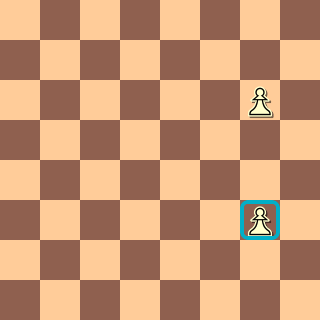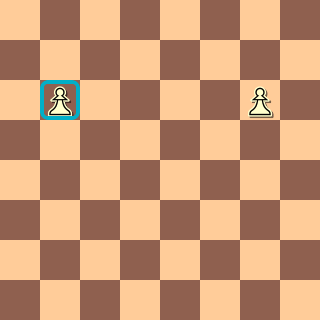`fliphorizontal Pg6` `flipvertical Pg6` `flip Pg6 ` [Pg6,Pb6] [Pg6,Pg3] [Pg6,Pg3,Pb6,Pc7,Pb3,Pf2,Pf7,Pc2]

`rotate90` has associated basic transforms corresponding to rotations of 0°, 90°, 180°, and 270°.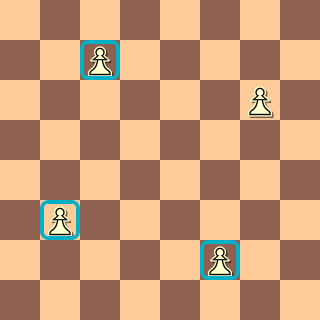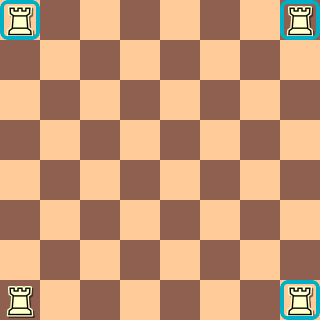`rotate90 Pg6` `rotate90 Ra1` ≡ [Pg6,Pc7,Pb3,Pf2] ≡ [Ra1,Rh1,Rh8,Ra8]

`rotate90` does a 4-fold transform on a position. When combined with `fliphorizontal` or `flipvertical` it is in fact equivalent to `flip`. For example:

```flip g6
≡  rotate90 fliphorizontal g6
≡  rotate90 [g6,g3]
≡  [g6,g3,b6,b7,b3,f2,f7,c2]```

Any transform can be applied recursively to the constituents of a filter. For example,

`flipvertical {Rg6 attacks k)`
is equivalent to
`{Rg6 attacks k} | {Rb6 attacks k}`
`rotate90 {Rg6 attacks k} `
is equivalent to
```{Rg6 attacks k}
| {Rc7 attacks k}
| {Rb3 attacks k}
| {Rf2 attacks k}```

Directions are also transformed:

`rotate90 ray up (R[g6,a1] k)`
is equivalent to
```ray up (R[g6,a1] k) |
ray left (R[c7,h1] k) |
ray down (R[b3,h8] k) |
ray right (R[f2,a8] k)```

## shift transforms

A shift transform shifts the squares in its single argument filter 0 or more times along some direction. There are three shift filters:
• shiftvertical
• shifthorizontal
• shift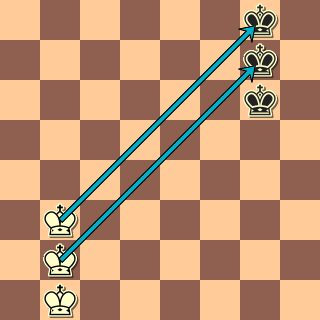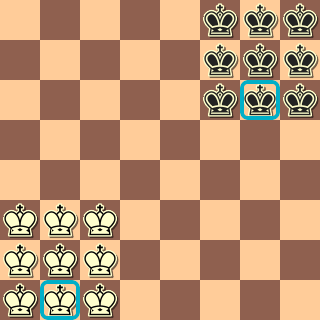`shifthorizontal {Kb1 kg6}` `shiftvertical {Kb1 kg6}` `shift {Kb1 kg6}` Kb1 kg6 | Ka1 kf6 | Kc1 kh6 Kb1 kg6 | Kb2 kg7 | Kb3 kg8 Kb1 kg6 | Kb2 Kg7 | Kb3 Kg8 Ka1 kf6 | Ka2 Kf7 | Ka3 Kf8 Kc1 kh6 | Kc2 Kh7 | Kc3 Kh8

`shiftvertical` shifts its argument 0 or more squares vertically:

```shiftvertical g6
≡ g1 | g2 | ... | g8
≡ g1-8```
. Likewise,
```shiftvertical [g2,g4]
≡ g1-8```
When a square is shifted off the board it normally disappears. Piece designators with empty square sets eliminate the entire transform:
```shiftvertical {Kb1 kg6}
≡
{Kb1 Kg6}
| {Kb2 Kg7}
| {Kb3 Kg8}```
There is no downward shift of `kg6` because doing so would eliminate the b1 square for the K.

`shifthorizontal` works likewise.
shift is equivalent to `shifthorizontal shiftvertical`. Using the example above:

```shift {Kb1 kg6}
≡  shifthorizontal shiftvertical {Kb1 kg6}
≡  shifthorizontal {Kb1 kg6} or shifthorizontal {Kb2 kg7} or shifthorizontal {Kb3 kg8}
≡
{Kb1 kg6} | {Ka1 kf6} | {Kc1 kh6} |
{Kb2 kg7} | {Ka2 kf7} | {Kc2 kh7} |
{Kb3 kg8} | {Ka3 kf8} | {Kc3 kh8}```
This also means that
`shift Ka2  ≡  K`

### wraparound

As a special rule, `shiftvertical` does not alter a file of 8 squares:
```shiftvertical {Kd1-8 Ba2}
≡
{Kd1-8 Ba2} |
{Kd1-8 Ba3} |
{Kd1-8 Ba4} |
...
{Kd1-8 Ba8} |
{Kd1-8 Ba1}```
which turns out to be equivalent to
`{Kd1-8 Ba1-8}`
. But
```shiftvertical {Kd2-8 Ba2}
≡
{Kd2-8 Ba2} |
{Kd3-8 Ba3} |
{Kd4-8 Ba4} |
{Kd5-8 Ba5} |
{Kd6-8 Ba6} |
{Kd7-8 Ba7} |
{Kd8 Ba8} |
{Kd1-7 Ba1}```
which is entirely different. Similarly, `shifthorizontal` does not change ranks with 8 squares:
```shifthorizontal {Ka-h2 Ba4}
≡   {Ka-h2 Ba-h4}```
The `shift` transform doesn't change full ranks or files in the direction of its shift:

```shift {Ka-h2 Ba4}
≡
{Ka-h2 Ba-h4} |
{Ka-h3 Ba-h5} |
{Ka-h4 Ba-h6} |
{Ka-h5 Ba-h7} |
{Ka-h6 Ba-h8} |
{Ka-h1 Ba-h3}```
Note that any transform applied to a piece designator without an explicit square qualifier leaves the piece designator unchanged:
```shift K
≡  K```
and likewise
```flip K
≡  K```

## The flipcolor and reversecolor transforms

The `flipcolor` transform applied to filter is the `or` (or | in the case of a set filter argument) of filter with the new filter formed from the filter as follows:

• the colors of any piece designators in `filter` are changed;
• `wtm` is changed to `btm` and vice versa;
• `player white` is changed to `player black` and vice versa;
• `elo white` is changed to `elo black` and vice versa
• `result 0-1` is changed to `result 1-0` and vice versa
• All piece designators in filter are reflected about the horizontal bisector of the board.
```flipcolor Ba1
≡
Ba1 | ba8```
Similarly,
```flipcolor {wtm result 1-0 [Pk]a-h2}
≡
{wtm result 1-0 [Pk]a-h2}
or {btm result 0-1 [pK]a-h7}```

The `reversecolor` transform is the same as the `flipcolor` transform except that the identity transform is not allowed. Instead, the colors are reversed and the board flipped. Because `reversecolor` can always be done by hand in the CQL file itself, it is normally only used at the command line using `-reversecolor`

For example,

```reversecolor Ba1
≡
ba8```

## The rotate45 transform and the cyclic group of order 8

`rotate45` is an unusual transform in that it is not allowed to operate on individual squares. Imagine an old-fashioned analogue clock. We will only focus on the minute hand of that clock.

Moving the minute-hand counterclockwise 45 degrees is the same as moving it back in time by 7.5 minutes. I will call this transform the unit counterclockwise transform. If we apply the unit counterclockwise transform twice, then the minute hand goes back by 15 minutes. If we apply it 8 times, then the minute hand stays unchanged.

Therefore, if we are only allowed to apply the unit counterclockwise transform to the minute hand, there are exactly 8 possible transformations of the minute hand, corresponding to moving the hand back 7.5 minutes, 15 minutes, 22.5 minutes, 30 minutes, 37.5 minutes, 45 minutes, 52.5 minutes, and leaving it unchanged.

These 8 transformations are the so-called cyclic group of order 8.

The transformations apply to a CQL filter as well, as long as the filter doesn't name any specific squares. All such a transformation does is change directions. A direction corresponds to a position of the minute hand: up is 12:00; down is 12:30; northeast is 12:07:30; and so on. So the unit counterclockwise transformation transforms up into northwest; northwest into left; left into southwest; and so on.

Thus, `rotate45 filter` takes the `or` (or the | in the case of a set filter) of all the elements of the order 8 cyclic group applied to the filter. For example,

```rotate45 up 1 K
≡
up 1 K
| northwest 1 K
| left 1 K
| southwest 1 K
| down 1 K
| southeast 1 K
| right 1 K
| north east 1 K```
Note that this is exactly the King's field: the set of squares adjacent to the King. However, we cannot of course apply `rotate45` to a filter that names any particular square, because a particular square cannot be rotated 45 degrees. Thus,
`    rotate45 Kd3 ; ERROR`

## Transforms with ranges

If followed by a range, any transform becomes a numeric filter and counts the number of transforms of the argument filter that match a position. For example,

`    shift 10 20 [Pp]a4`
would match positions with between 10 and 20 pawns on the board (although of course it would be much slower than simply writing `Pp 10 20`.

This gives us a compact way to check, say, if at some point in the game all 4 corners of the board are visited by a rook:

```    initial
rotate90 4
find Ra1```
Here, find Ra1 is true if at the current position or in the future, a white rook is on a1. The 4 rotations of this filter are:
```rotate90 find Ra1
≡
{find Ra1}
| {find Rh1}
| {find Rh8}
| {find Ra8}```
Here, however, the `4` following the `rotate90` means that each of the 4 elements of the rotation group must match. Thus

```rotate90 4 find Ra1
{find Ra1
find Rh1
find Rh8
find Ra8}```
That is, each of the 4 clauses must be true. (Recall that when a sequence of filters is enclosed in braces each constituent filter must match). This idea is used to give a compact statement of the problem of finding games in which the same rook visits all 4 corners of the board.

### Using ranges to count occurrences of configurations

As another example, consider a configuration where a white queen and a black queen are separated by a single square, with a piece on that square. For example, `Qd4 ne4 qf4` is an example of such a configuration. There are eight possible orientations of the queens: the Q can be below the q, or northeast of the q and so on.

How can we find all games in which at least 5 of these configurations occur, sorted by the number of the configurations?

The simplest way is first to express one of valid configurations, say the one we listed above where the q is two squares to the right of the Q and a piece is between them. This configuration turns out to be:

`q&right 1 [Aa]&right 1 Q`

Written with braces, this expression is:

```    q &
{right 1
{[Aa]&
{right 1 Q}}}```
Suppose the position is as in our example: `Qd4 ne4 qf4`. Will it match?

Well, Q is d4, so this is:

```    q &
{right 1
{[Aa]&
{right 1 d4}}}```
But `right 1 d4` is e4, so this is:
```    q &
{right 1
{[Aa]&e4}```
There is a `[Aa]` on e4, namely a black knight, so the last expression is just `e4`
```    q &
{right 1 e4}
≡
q&f4```
So this does in fact match our test position. And this should make clear why it would in fact match the positions where the `Q` is two squares right of the `q` separated by a piece. But the key thing to notice is that the `right` direction can be any of the eight compass directions. Thus, to count configurations where 5 of the eight configurations occur, we stick a `rotate45` in front and sort
```   sort rotate45 5 8
find q&right 1 [Aa]&right 1 Q```
This example is taken from Qq-rotations.cql.

If you just want to test for rotations by multiples of 90 degrees, and not the 45 degree rotations, this code might be easier to follow, which tests for rotations of the theme configuration by multiples of 90 degrees: it names explicit squares and does not use direction operators.

```     rotate90 4
find shift {Qd4 [Aa]e4 qf4}```
However, in general `shift` is much slower and less flexible than using the direction operators . This example code is in Qq-rotations-90-degree.cql

## Things to watch out for when using transforms

Using transforms can create code that is concise and easy to understand compared to using `square` and `piece`. But there are some pitfalls:
1. transforms create code that is less flexible and more difficult to generalize than using `square` and `piece`. Counting and sorting can be more difficult.
2. transforms can make the automatically generated comments more difficult to understand
3. transforms, especially shift transforms, can be slower than using `square ` or `piece`.